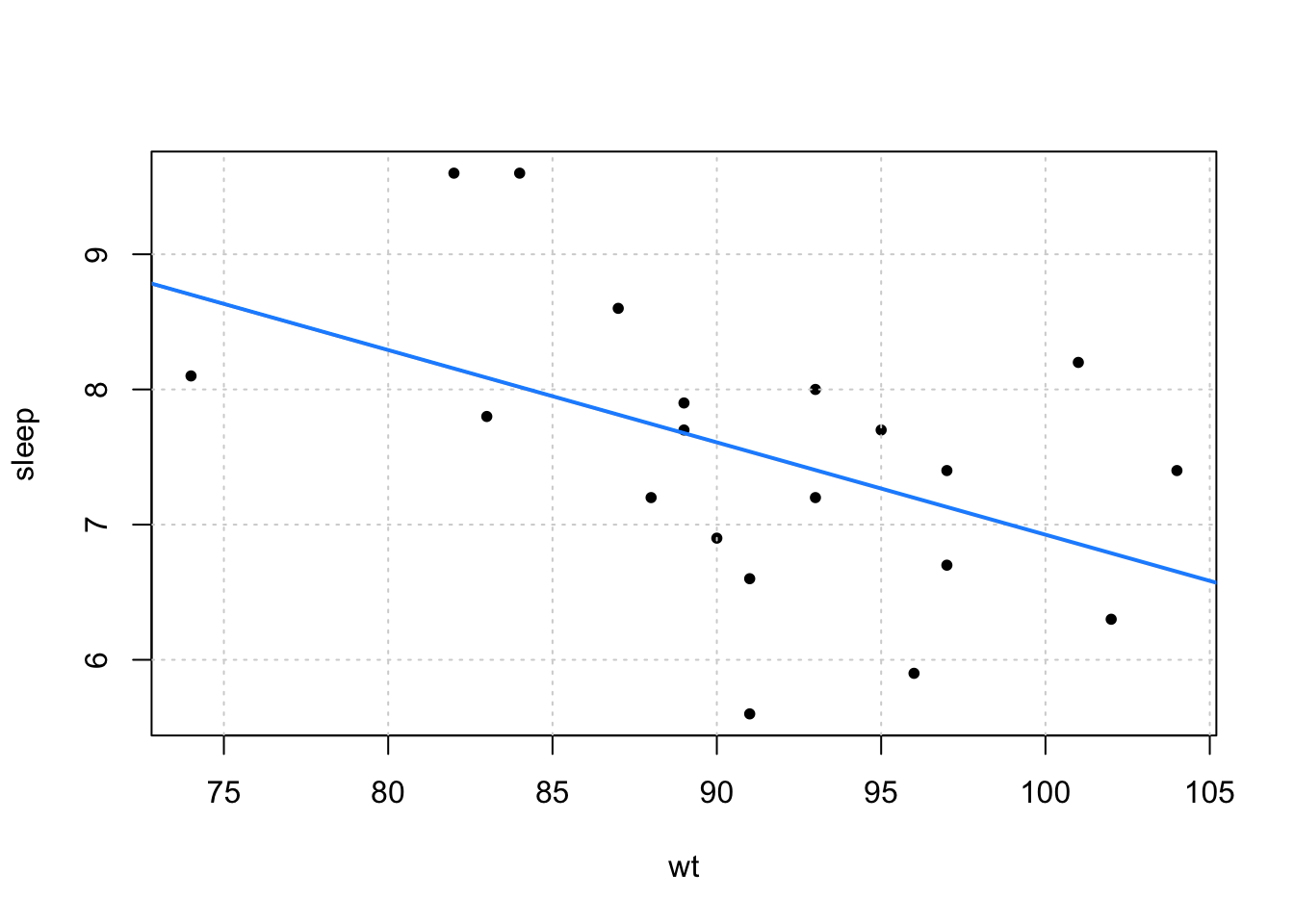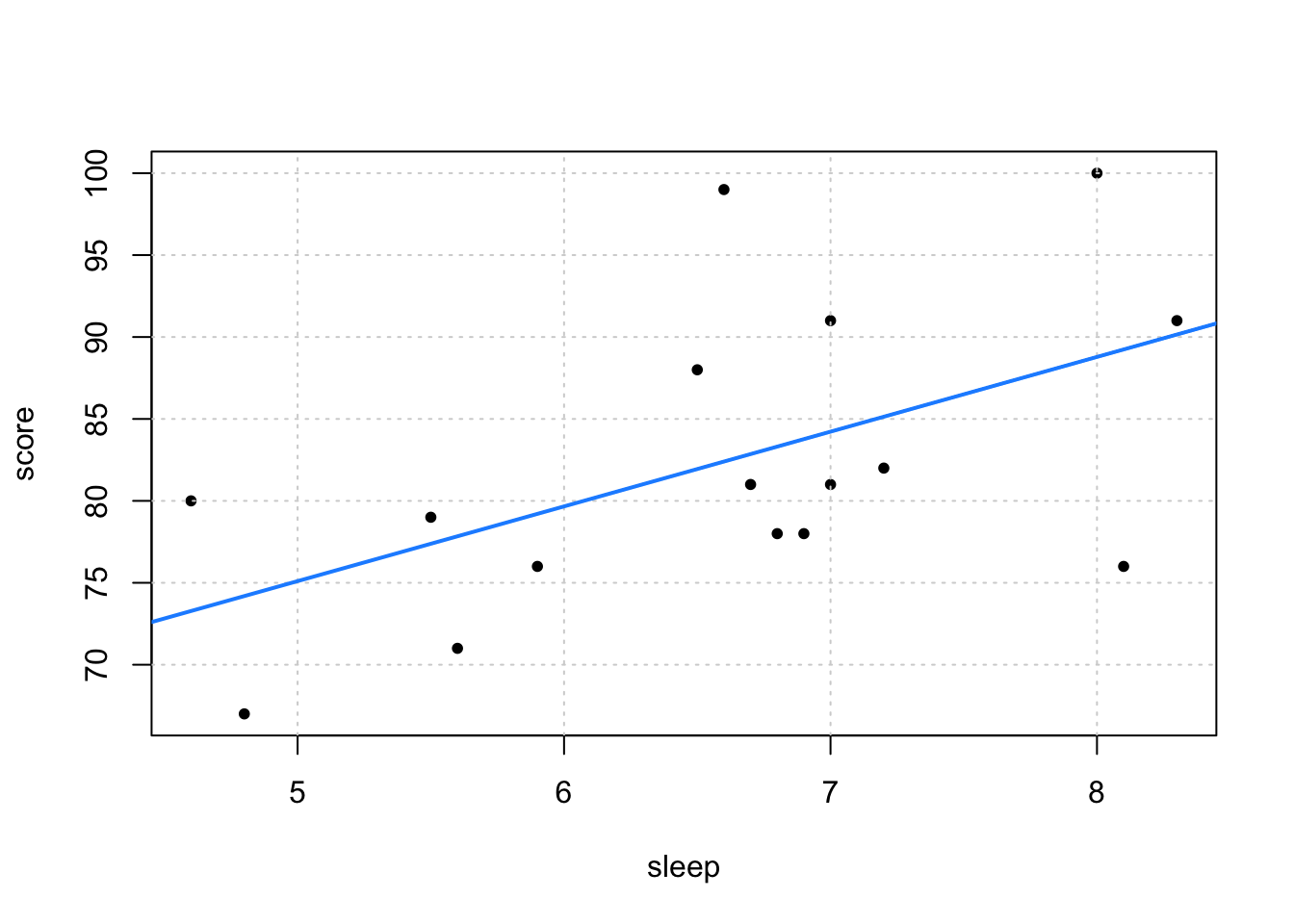# Exercise 1The above (simulated, and not the same as the previous homework) data shows the relationship between sleep (in hours) and weight (in kilograms) of a random sample of adult males on a particular night. A simple linear regression model was fit to this data. The fitted line is added to the above plot.

##
## Call:
## lm(formula = sleep ~ wt, data = sleep_wt_data)
##
## Residuals:
##     Min      1Q  Median      3Q     Max
## -1.9405 -0.5596 -0.0905  0.6341  1.5812
##
## Coefficients:
##             Estimate Std. Error t value Pr(>|t|)
## (Intercept) 13.75851    2.71887    5.06 8.15e-05 ***
## wt          -0.06833    0.02969      NA       NA
## ---
## Signif. codes:  0 '***' 0.001 '**' 0.01 '*' 0.05 '.' 0.1 ' ' 1
##
## Residual standard error: 0.951 on 18 degrees of freedom
## Multiple R-squared:  0.2274, Adjusted R-squared:  0.1845
## F-statistic: 5.297 on 1 and 18 DF,  p-value: 0.03352

Some evil professor has hacked R and ruined the output from the summary() function. Use what information is provided to carry out the test

$H_0: \beta_1 = 0 \quad \text{vs} \quad H_1: \beta_1 \neq 0$

Report:

• The value of the test statistic
• The p-value of the test
• Provide a single line of R code used to perform this calculation.
• A decision using $$\alpha = 0.05$$

# Exercise 2

Using only the information provided in Exercise 1, create 95% confidence intervals for $$\beta_0$$ and $$\beta_1$$.

# Exercise 3The above (simulated, and not the same as the previous homework) data shows the relationship between exam scores and sleep (in hours) for a random sample of students in a large statistics course. A simple linear regression model was fit to this data. The fitted line is added to the above plot.

• $$(\bar{x}, \bar{y}) = (6.59375, 82.375)$$
• $$n = 16$$
• $$S_{xx} = 17.869375$$
• $$S_{yy} = 1273.75$$
• $$S_{xy} = 81.5375$$
• $$RSS = 901.6964779$$

Use the above statistics to calculate:

• A 99% confidence interval for the mean exam score of students who sleep 7 hours.
• A 99% prediction interval for the exam score of a student who sleeps 7 hours.

# Exercise 4

The following three plots show:

• The data and fitted regression for the Orange data in R. Here we are using the circumference of oranges as the response and the age of tree as the predictor.
• A fitted versus residuals plot
• A normal qq-plot